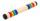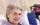Hiker

Hiker went half of trip the first day, the third of trip the second day and remaines 15 km. How long trip he planned?

Result

s =  90 km

Solution:x-x/2-x/3 = 15

x = 90

x = 90

Calculated by our simple equation calculator.

Leave us a comment of this math problem and its solution (i.e. if it is still somewhat unclear...):Be the first to comment!To solve this verbal math problem are needed these knowledge from mathematics:

Need help calculate sum, simplify or multiply fractions? Try our fraction calculator. Do you have a linear equation or system of equations and looking for its solution? Or do you have quadratic equation? Do you want to convert length units?

Next similar math problems:

1. BarHalf of the bar is green, the third is yellow and the remaining 40cm is red. How long is a bar?
2. SeamstressThe seamstress cut the fabric into 3 parts. The first part was the eighth fabric, the second part was three-fifths of the fabric and the third part had a length of 66 cm. Calculate the original length of the fabric.
3. One thirdIf 3/5 is 360, how much is 1/3?
4. UN 1If we add to an unknown number his quarter, we get 210. Identify unknown number.
5. EquationSolve equation and check the result: 1.4x - 3/2 + x - 9,8 = x + 0,4/3 - 7 + 1,6/6
6. Cupcakes 2Susi has 25 cupcakes. She gives 4/5. How much does she have left?
7. Forest nurseryIn the forest nursery after winter, they found that 1/10 stems died out of them. For them, they land 193 new spruces. How many spruces are in the forest nursery?
8. Equation 15Solve equation with variables on both sides:
9. Equation with xSolve the following equation: 2x- (8x + 1) - (x + 2) / 5 = 9
10. Equation with fractionsSolve equation: ? It is equation with fractions.
11. Find xSolve: if 2(x-1)=14, then x= (solve an equation with one unknown)
12. Simple equation 6Solve equation with one variable: X/2+X/3+X/4=X+4
13. PearsThere were pears in the basket, I took two-fifths of them, and left six in the basket. How many pears did I take?
14. FamilyMom is 39 years old. Karl is eight years and Paul 4 years. For many years mother would old just like two children together?
15. FractionsThree-quarters of an unknown number are 4/5. What is 5/6 of this unknown number?
16. EqnSolve equation with fractions: 2x/3-50=40+x/4
17. Eq-fracSolve the following equation with fractions: h + 1/3 =5/3